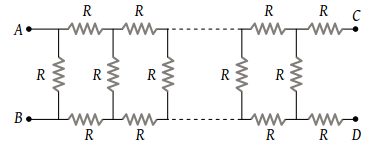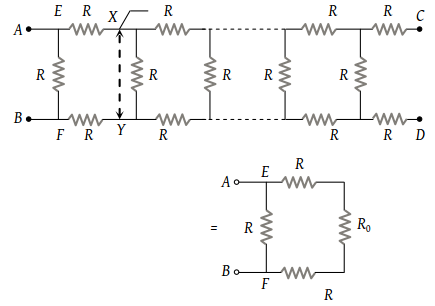In the figure, the value of resistors to be connected between C and D so that the resistance of the entire circuit between A and B does not change with the number of elementary sets used is(1) R

(2) $R\left(\sqrt{3}-1\right)$

(3) 3 R

(4) $R\left(\sqrt{3}+1\right)$

(2) Cut the series from XY and let the resistance towards right of XY be ${R}_{0}$ whose value should be such that when connected across AB does not change the entire resistance. The combination is reduced to as shown below.The resistance across $EF={R}_{EF}=\left({R}_{0}+2R\right)$

Thus ${R}_{AB}=\frac{\left({R}_{0}+2R\right)R}{{R}_{0}+2R+R}=\frac{{R}_{0}R+2{R}^{2}}{{R}_{0}+3R}={R}_{0}$

$⇒{R}_{0}^{2}+2R{R}_{0}-2{R}^{2}=0⇒{R}_{0}=R\left(\sqrt{3}-1\right)$

Difficulty Level:

• 11%
• 61%
• 11%
• 18%